# Number sense

Here you will learn about number sense, including what it is and different ways to develop it.

Students will first learn about number sense from the beginning of their experiences with numbers and they continue to build on this knowledge throughout all experiences in math.

## What is number sense?

Number sense is the ability to think flexibly and critically about numbers and their operations.

Someone with a strong number sense can…

• Solve or make reasonable estimates using mental math.
• Represent numbers or solve operations in more than one way.
• Make connections between solving strategies.

Number sense is not a “check the box” kind of skill. Each student lies somewhere on the spectrum of number sense, and with each mathematical experience there is an opportunity to build a deeper understanding or “sense of number.”

Number sense is embedded into any work with numbers and operations. This page will specifically cover whole numbers and the operations of addition and subtraction.

In young learners, building number sense around addition and subtraction may look like:

• Briefly showing students dominoes and asking them to describe how many they saw.
For example,
Show the image below for 3 seconds (so students cannot count the dots).

Then ask them to share what they saw.

• Ask students to represent the number 8 in as many ways as they can. Then let students explain and compare their representations with others.

As students progress in their number sense and are ready to begin operating with numbers, activities may look like:

For example,

• Ask first grade students to compare the numbers 23 and 33 in more than one way. Then let students explain their comparisons with others. Then ask students how they could apply other students’ strategies to compare 33 and 43.

• Ask 2 nd grade students to subtract 83-59 mentally. Then ask students to share their thinking, while dictating their strategy on the board. Prompt students to make connections between the strategies they see being shared.

• Ask 3 rd grade students what number bond can help them solve 400-150. Then ask them how the same number bond could also help them solve 401-151 and 399-149. Encourage students to journal about their strategies or share them with other classmates.

### What is number sense?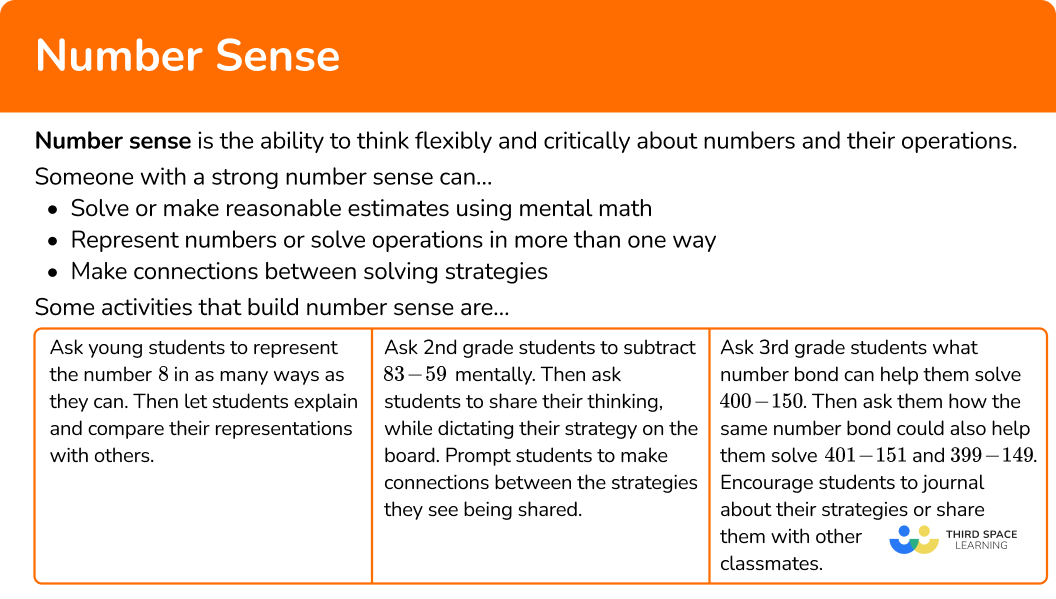## Common Core State Standards

How does this relate to 1 st grade math, 2 nd grade math and 3 rd grade math?

• Grade 1 – Numbers and Operations in Base 10 (1.NBT.B.2)
Understand that the two digits of a two-digit number represent amounts of tens and ones.

• Grade 2 – Numbers and Operations in Base 10 (2.NBT.B.5)
Fluently add and subtract within 100 using strategies based on place value, properties of operations, and/or the relationship between addition and subtraction.

• Grade 3 – Numbers and Operations in Base 10 (3.NBT.A.1)
Use place value understanding to round whole numbers to the nearest 10 or 100.

• Grade 3 – Numbers and Operations in Base 10 (3.NBT.A.2)
Fluently add and subtract within 1000 using strategies and algorithms based on place value, properties of operations, and/or the relationship between addition and subtraction.

## How to develop number sense

In order to develop number sense:

1. Create mental strategies for thinking about numbers and operations.
2. Practice representing strategies in more than one way.
3. Share strategies and listen to the strategies of others, comparing and contrasting.

## Number sense examples

### Example 1: understanding tens and ones

How many tens and ones are in the number 27?

1. Create mental strategies for thinking about numbers and operations.

Picture 27 hearts in your head for a moment… How do you see them?

Maybe you see a straight line of 27…

Maybe you see 27 in groups, like in five frames…

Maybe you see 27 in groups, like in ten frames…

There is not a “wrong” way to picture 27, but notice which ways make it easier to understand 27 as a quantity.

2Practice representing strategies in more than one way.

Now, think about different ways to show 27 with base 10 blocks.

Maybe you show 27 ones…

Maybe you show 1 ten and 17 ones…

Maybe you show 2 tens and 7 ones…

There are many ways to group 27, but notice which ways make it easier to understand 27 as a quantity.

3Share strategies and listen to the strategies of others, comparing and contrasting.

Looking at all the strategies above, what is similar? What is different? Did you think of 27 in another way?

### Example 2: add within 100

Solve 33 + 48.

Maybe you started at 33 and counted up 48 \text{:}

34, \, 35, \, 36, \, 37, \, 38, \, 39, \, 40, \, 41, \, 42….

Maybe you grouped the tens and the ones:

30 + 40 = 70 , and 3 + 8 = 11 .

So now you can add 70 + 11.

Maybe you broke apart 33 to make a ten:

33 + 48

= (31 + 2) + 48

= 31 + (2 + 48)

= 31 + 50

Grouping the 2 + 48 together makes 50, so now you can solve 31 + 50.

There are many ways to mentally solve 33 + 48, but notice which makes the most sense to you.

One way to represent 33 + 48 is with base 10 blocks.

Another way is to use a hundreds chart.

You can also create hops on a number line:

There are many ways to represent 33 + 48, but notice which makes the most sense to you.

Looking at all the strategies above, what is similar? What is different? Would you solve 33 + 48 a different way?

### Example 3: subtract within 100

Solve 84 - 68.

Take 68 away from 84 in your head… How did you subtract the numbers?

Maybe you started at 68 and counted up 84 \text{:}

69, \, 70, \, 71, \, 72, \, 73, \, 74, \, 75, \, 76, \, 77….

Maybe you started at 68 and counted up by groups of ones and tens:

From 68 to 70 is 2.

From 70 to 84 is 14 more.

So the distance from 68 to 84 is 16.

Maybe you broke apart 68 to subtract each place value:

84 - 60 = 24

24 - 8 = 16

Something to think about: There are many ways to mentally solve 84 - 68, but notice which makes the most sense to you.

One way to represent 84 - 68 is with base 10 blocks.

Another way is to use a hundreds chart.

You can also create hops on a number line:

There are many ways to represent 84 - 68, but notice which makes the most sense to you.

Looking at all the strategies above, what is similar? What is different? Would you solve 84 - 68 a different way?

## How to develop specific number sense strategies

In order to develop specific number sense strategies:

1. Decide if making \textbf{10} or using number bonds can help you solve.
2. Solve with your strategy and explain why it works.

### Example 4: subtract within 20

Solve 16 - 7.

Think about what number plus 7 is equal to 16 to complete the number bond.

Since 9 completes the number bond, it is the difference between 16 and 7.

16 - 7 = 9.

### Example 5: add within 100

Solve 34 + 37.

It is not always easy to remember larger number bonds, but you can make 10.

Think about how you can regroup part of 34 with 37 to make a multiple of 10.

For example,

34 + 37

= (31 + 3) + 37

= 31 + (3 + 37) \quad *You can regroup 3 to go with 37.

= 31 + 40

= 71

So, 34 + 37 = 71.

### Example 6: subtract within 1,000

Solve 300 - 150.

Sometimes you can use smaller number bonds, to help solve operations with larger numbers.

Think about what number plus 15 is equal to 30 to complete the number bond.

Since 15 completes the number bond, the difference between 30 and 15 is 15. Since 300 and 150 are 10 times larger, their difference is also 10 times larger.

So, 300 - 150 = 150.

### Teaching tips for number sense

• Do your best to embed number sense activities into all math lessons and through all math centers, math skills and math problems. This does not require extensive extra planning – instead always look for ways for students to solve problems in multiple ways, explain their problem solving (written or orally) and critique the strategies of others.

• Many activities will naturally lend themselves to building number sense, particularly activities with real-life contexts, the use of hands-on manipulatives, and a classroom emphasis on problem-solving. While not always appropriate, worksheets that encourage students to solve in more than one way or analyze the thinking of others can also be useful.

### Easy mistakes to make

• Thinking that children need to be a certain year old to develop number sense
Even before students can formally use numerals or other number symbols, they can develop their sense of number. Some activities for pre-k students might include subitizing (recognizing the number of objects without counting), identifying more and less when comparing two groups of objects or learning to count using number words.

• Teaching algorithms too quickly
Introducing algorithms before students have had time to explore a topic and grapple with their own ideas can eliminate a student’s motivation, creativity, and ownership and encourage memorization of rules over understanding.

While there is no hard and fast rule as to how to progress a topic, be mindful in giving students time to develop ideas and remember that building foundational understanding takes time.

• Requiring students to use specific number sense strategies
Unless directed by your state standards to do so, it is not necessary to insist that students use a certain strategy or ask students to memorize a strategy. While this is often done with good intentions, it is similar to asking students to memorize or use an algorithm too quickly.

The best way to promote the use of number sense within the classroom is to use activities that allow students to solve in more than one way and consistently ask students to talk about their strategies. It is also helpful to promote a growth mindset and help students see the value in admitting to and learning from their mistakes.

### Practice number sense questions

1) Which choice is NOT equal to 36?

3 tens and 6 ones26 ones and 1 ten6  ones and 30 tens2 tens and 16 ones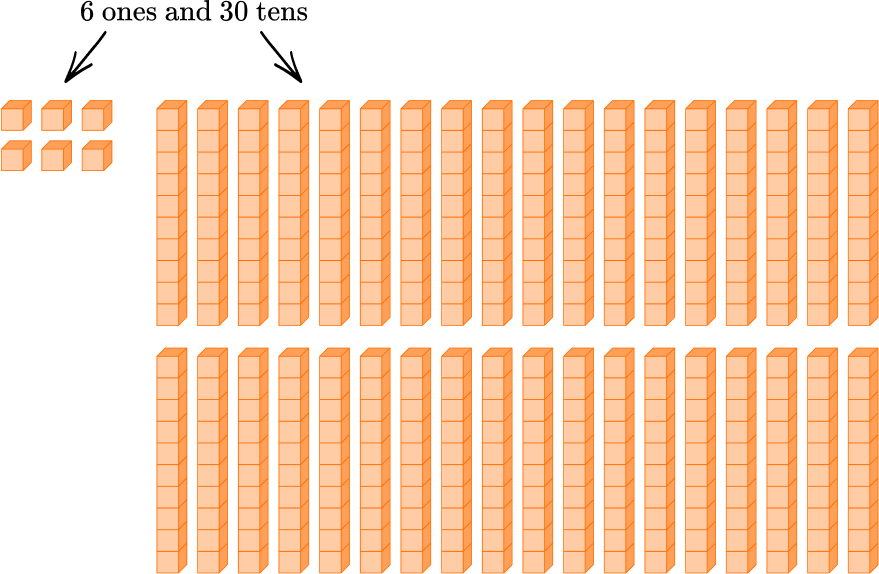The model above shows 6 ones and 30 tens, which is NOT equal to 36.

It is equal to 306.

2) Solve 18-11.

67119There are many ways to solve 18-11. Two ways are with a model and by using a number bond.

Show the tens and ones in 18 with a model and then subtract 11 \text{:}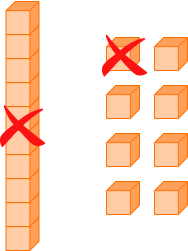Use a number bond to solve.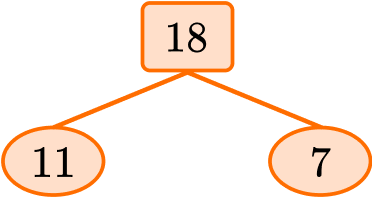Both ways show that 18-11 = 7.

3) Solve 46 + 19.

27555665There are many ways to solve 46 + 19. Two ways are with a model and by making 10.

Show the tens and ones with a model and then combine them: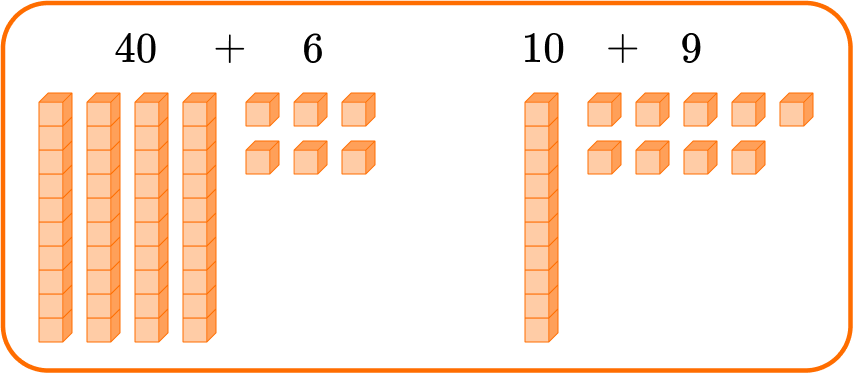Regroup 46 to make a multiple of 10.

46 + 19

= (45 + 1) + 19

= 45 + (1 + 19)

= 45 + 20

= 65

4) Which strategy does NOT show 18 + 27?

“18 = 3 + 15, so I add 27 + 3 = 30 and then 30 + 15.”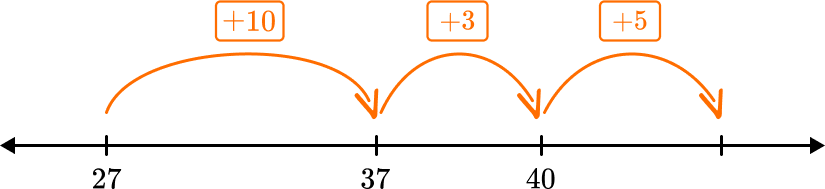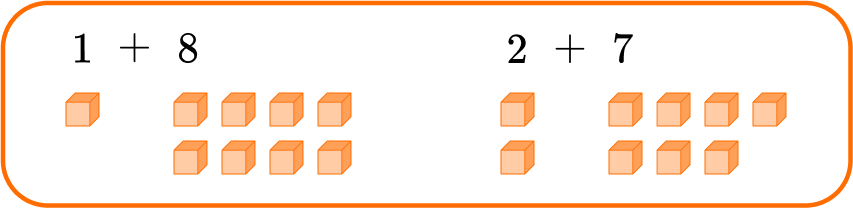“I started at 27 and counted up 18.”This model shows 1 + 8 + 2 + 7 which is NOT the same as 18 + 27.A correct model for 18 + 27 is shown below.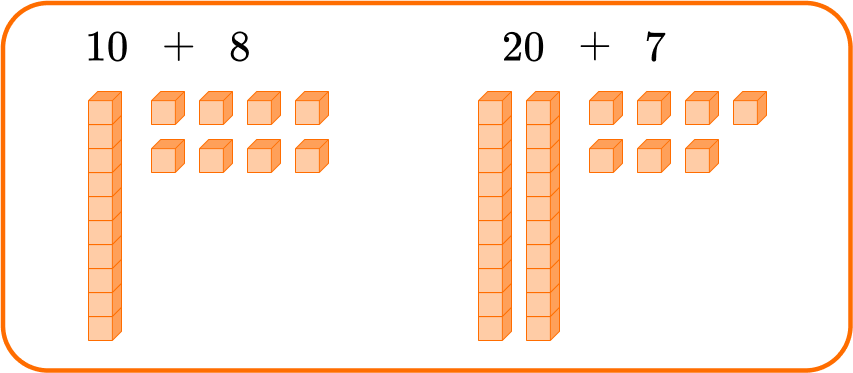*Note: Other models can also be used to show 18 + 27, but all correct models show a total of 45.

5) Which strategy does NOT show 35 + 26?

“26 = 5 + 21, so I add 35 + 5 = 30 and then 30 + 21.”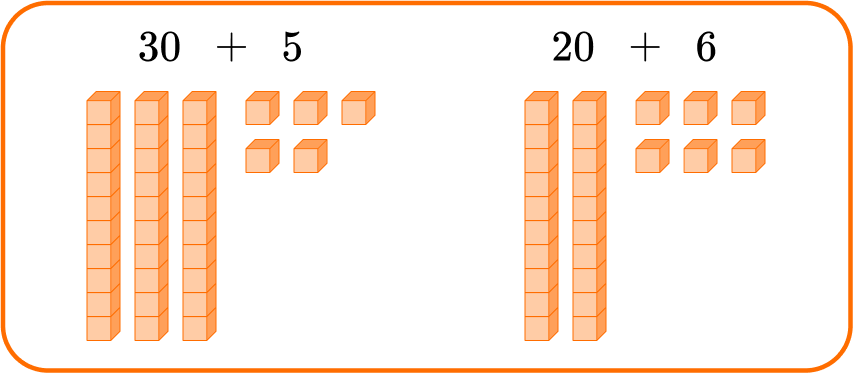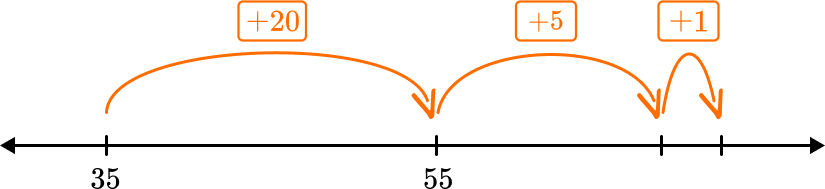“I started at 35 and counted up 26.”There is a mistake in this explanation:

“26 = 5 + 21, so I add 35 + 5 = 30 and then 30 + 21.”

35 + 5 = 40, so the correct strategy is:

35 + 26

= 35 + (5 + 21)

= (35 + 5) + 21

= 40 + 21

*Note that this strategy, making 10, can also be used with different numbers.

6) Solve 600-200.

4040020200There are many ways to solve 600-200. Two ways are with a model and by using a number bond.

Show the hundreds in 600 with a model and then subtract 200 \text{:}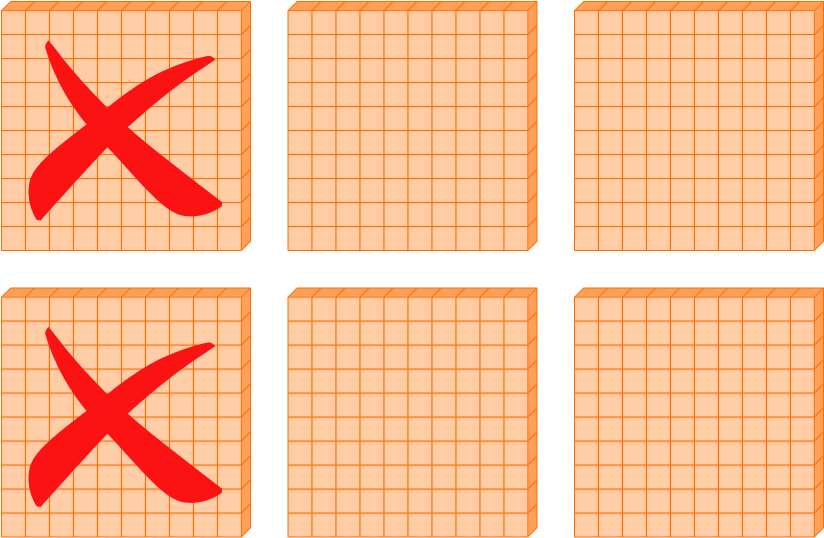Use a number bond to solve.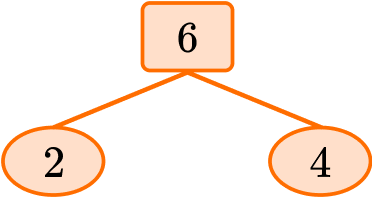2 + 4 = 6, so 200 + 400 = 600, since the numbers in the bond are 100 times larger.

Both ways show that 600-200 = 400.

## Number sense FAQs

Why are number sense skills important?

Success in mathematics depends on a deep understanding of numbers. How students learn math can impact the level of this understanding.

A focus on developing number sense in elementary school promotes flexible thinking around whole numbers, fractions and decimals. This type of knowledge helps students understand concepts more deeply and encourages creative approaches to problem solving.

This is particularly important as math topics become more abstract in middle school and high school. Students who have greater number sense are often more successful at applying what they know to new and more complex mathematics.

How do math facts connect to number sense?

For younger students, much of their development of number sense comes from activities that involve math facts.

This includes (but is not limited to) opportunities to solve with models and drawings, solving real-world problems that involve basic math facts, solving math facts mentally and sharing and critiquing solving strategies with others.

For older students, math facts can be a tool utilized to solve complex problems more efficiently.

## Still stuck?

At Third Space Learning, we specialize in helping teachers and school leaders to provide personalized math support for more of their students through high-quality, online one-on-one math tutoring delivered by subject experts.

Each week, our tutors support thousands of students who are at risk of not meeting their grade-level expectations, and help accelerate their progress and boost their confidence.